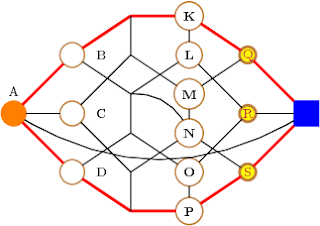## Tuesday, August 18, 2009

### Several options for the \Vertex macro

UPDATE: This example no longer works with the latest versions of tkz-graph and tkz-berge. For an updated version see this post at my new blog.\begin{tikzpicture}
\SetVertexNormal[LineColor=brown]
\Vertex[x=0,y=2.5,style=orange,LabelOut=true,Lpos=90]{A}
{\tikzstyle{every node} = [node distance=1.5cm]
\Vertices[x=1.5,y=4,dir=\SO,LabelOut=true,Ldist=5pt]{B,C,D}}
\Vertices[x=3,y=5,dir=\SO,style={shape=coordinate}]{E,F,G,H,I,J}
\Vertices[x=4.5,y=5,dir=\SO,style={font=\bfseries}]{K,L,M,N,O,P}
{\tikzstyle{every node} = [node distance=1.5cm]
\Vertices[x=6,y=4,dir=\SO,
style={line width=2pt,
inner sep=0pt,
text=purple,
fill=yellow,
minimum size=12pt}]{Q,R,S}}
\Vertex[x=7.5,y=2.5,style={shape=rectangle,blue}]{T}
\Edges[color=red,lw=2pt](A,B,E,K,Q,T,S,P,J,D,A)
\Edges(B,G,L,R,T)
\Edges(D,H,O,R)
\Edges(A,C,F,M,Q)
\Edges(C,I,N,S)
\foreach \x/\y in {E/F,G/H,I/J,K/L,M/N,O/P}
{\Edge(\x)(\y)}
\Edge[style={bend left}](G)(N)
\Edge[style={bend right}](A)(T)
\end{tikzpicture}

Benoît said...

Thx it helped me a lot

M. Tamer Özsu said...

I am having a trouble running your code. It gives me the following error

Argument of \Vertices@NoStar has an extra }.

\par
l.9 ...=4,dir=\SO,LabelOut=true,Ldist=5pt]{B,C,D}}

on line

\Vertices[x=1.5,y=4,dir=\SO,LabelOut=true,Ldist=5pt]{B,C,D}}

I have cut-and-pasted the code and enclosed it within

\documentclass{article}
\usepackage{tkz-berge}
\begin{document}

\end{document}

Rafael said...

@M. Tamer Özsu:

I can reproduce the problem, probably it is due to changes in tkz-berge. I'll look into it and post an update. Thanks for your report!

M. Tamer Özsu said...

Thanks. While you are at it, I wonder if I can ask another question. Is there a way to have a node with label both inside it and outside? I want to have a node identifier inside the node and the actual label above it, but have not been able to figure out if this can be done.

Rafael said...

I have updated another post in my new blog, see: http://graphtheoryinlatex.wordpress.com/2011/01/21/doing-more-with-vertices/, showing one way to have multiple labels on one vertex.